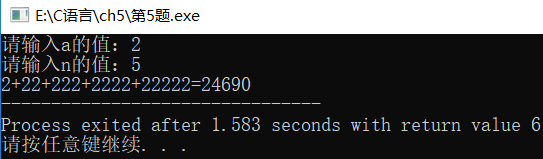# C 代码实现：

``````#include <stdio.h>
#include <math.h>

int main() {
int S,a,n,t;
int i,j;

printf("请输入a的值：");
scanf("%d", &a);

printf("请输入n的值：");
scanf("%d", &n);

if(n<=1){
printf("n 的值必须大于 1");
return 0;//退出程序
}

S = a;
//printf("第1次：%d\n", a);
printf("%d", a);

for(i=1; i<n; i++){
t = 0;

for(j=i; j>=0; j--){
t = t+a*pow(10, j);
}

printf("+%d", t);
//printf("第%d次：%d\n", i+1, t);
S = S+t;
}

printf("=%d", S);

return 0;
}``````

# 运行结果：

``````请输入a的值：2

2+22+222+2222+22222=24690``````